# Image-based graphs

Last time I showed you the basics of using the new graph theory functionality in MATLAB R2015b. Today I want to talk about some functions I put on the File Exchange for making graphs from images. Those of you working with graph-based image analysis algorithms might find them useful. I would be very interested in receiving feedback on these functions.

The function imageGraph creates a graph representing the neighbor relationships for every pixel in an image with a specified size. imageGraph3 does the same thing for a 3-D image array.

The function binaryImageGraph creates a graph representing the neighbor relationships for every foreground pixel in a binary image. binaryImageGraph3 creates a graph from a 3-D binary image array.

The function plotImageGraph plots a graph created by imageGraph or binaryImageGraph with the graph nodes arranged on a pixel grid.

Finally, the function adjacentRegionGraph creates a graph from a label matrix defining image regions. The graph represents the region adjacency relationships.

### Contents

#### Example: Image graph with 8-connected pixels

Create a graph for a 480-by-640 image with 8-connected pixels.

g = imageGraph([480 640],8)

g =

graph with properties:

Edges: [1225442x2 table]
Nodes: [307200x3 table]



Graph nodes contain the x-coordinate, y-coordinate, and linear index of the corresponding image pixels.

g.Nodes(1:5,:)

ans =

x    y    PixelIndex
_    _    __________

1    1    1
1    2    2
1    3    3
1    4    4
1    5    5



Plot the image graph using plotImageGraph.

plotImageGraph(g)
axis([100 110 220 230])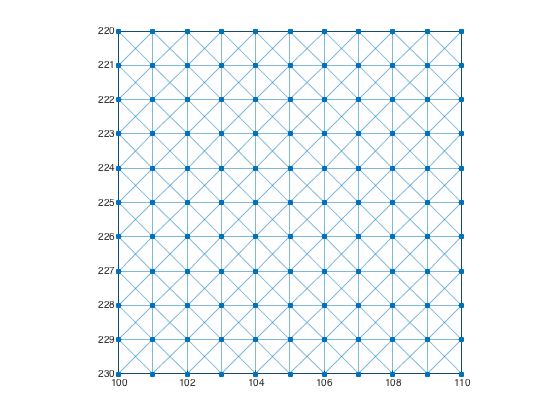#### Example: Image graph with 4-connected pixels

Create a graph for a 480-by-640 image with 4-connected pixels.

g = imageGraph([480 640],4)

g =

graph with properties:

Edges: [613280x2 table]
Nodes: [307200x3 table]


plotImageGraph(g)
axis([100 110 220 230])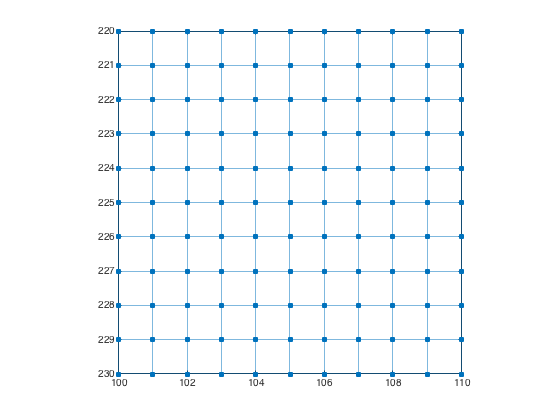#### Example: Image graph with special connectivity

Use a 3-by-3 connectivity matrix to create an image graph with 6-connected pixels. Each pixel is connected to its north, northeast, east, south, southwest, and west neighbors.

conn = [0 1 1; 1 1 1; 1 1 0];
g = imageGraph([480 640],conn);
plotImageGraph(g)
axis([100 110 220 230])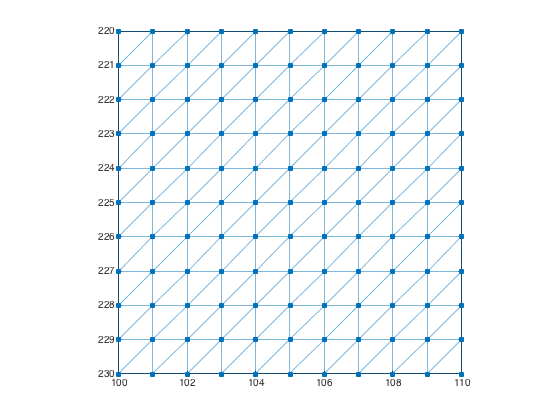#### Example: Binary image graph

Create a graph whose nodes are the foreground pixels of the binary image text.png (a sample image that is included with the Image Processing Toolbox).

bw = imread('text.png');
imshow(bw)
title('Original image')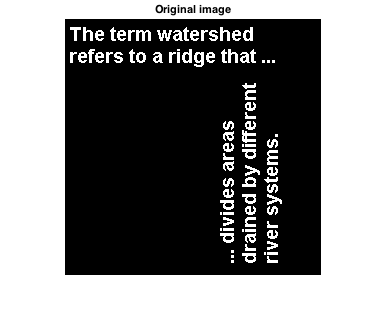g = binaryImageGraph(bw);
figure
plotImageGraph(g)
axis([60 85 30 45])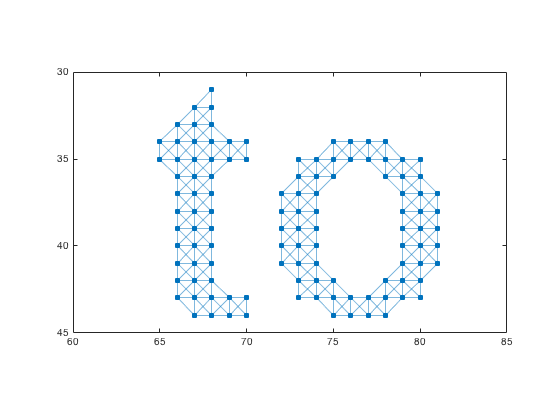#### Region Graphs

A label matrix defines a set of regions based on each unique element value in the matrix. For example, suppose you had the following label matrix:

L = [10 10 3 4.5 4.5; 10 10 3 4.5 4.5; 20 20 3 15 15; 20 20 3 15 15]

L =

10.0000   10.0000    3.0000    4.5000    4.5000
10.0000   10.0000    3.0000    4.5000    4.5000
20.0000   20.0000    3.0000   15.0000   15.0000
20.0000   20.0000    3.0000   15.0000   15.0000



This label matrix defines 5 regions:

• A 2-by-2 region in the upper left labeled with the value 10.
• A 2-by-2 region in the lower left labeled with the value 20.
• A one-pixel-wide vertical region in the middle labeled with the value 3.
• A 2-by-2 region in the upper right labeled with the value 4.5.
• A 2-by-2 region at the lower right labeled with the value 15.

The function adjacentRegionsGraph returns a graph with the same number of nodes as labeled regions. Edges in the graph indicate pairs of adjacent regions.

#### Example: Region graph

Compute an adjacent regions graph. Plot the graph, highlighting the nodes connected to the label 15.

g = adjacentRegionsGraph(L)

g =

graph with properties:

Edges: [6x2 table]
Nodes: [5x1 table]



The graph nodes contain the region labels.

g.Nodes

ans =

Label
_____

3
4.5
10
15
20



The graph edges contain the labels for each adjacent region pair.

g.Edges

ans =

EndNodes      Labels
________    __________

1    2        3    4.5
1    3        3     10
1    4        3     15
1    5        3     20
2    4      4.5     15
3    5       10     20



Plot the graph, capturing the GraphPlot object as an output argument.

gp = plot(g,'NodeLabel',g.Nodes.Label)

gp =

GraphPlot with properties:

NodeColor: [0 0.4470 0.7410]
MarkerSize: 4
Marker: 'o'
EdgeColor: [0 0.4470 0.7410]
LineWidth: 0.5000
LineStyle: '-'
NodeLabel: {'3'  '4.5'  '10'  '15'  '20'}
EdgeLabel: {}
XData: [0.0011 0.4051 -1.3642 1.3629 -0.4048]
YData: [0.0015 1.5564 -0.8540 0.8538 -1.5577]

Use GET to show all properties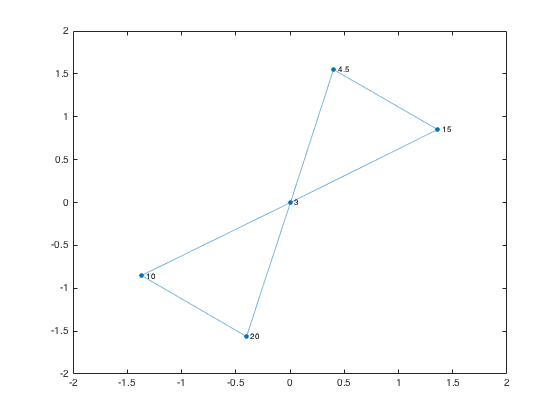Find the neighbors of the region labeled 15.

node_num = find(g.Nodes.Label == 15);
neighbors_15 = neighbors(g,node_num);
highlight(gp,node_num,neighbors_15)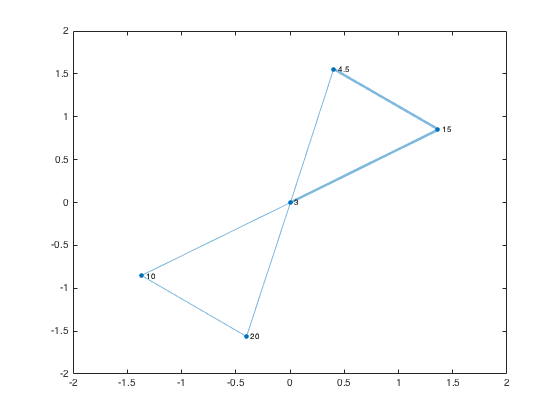Next time I plan to show you a graph-based maze solver ... that cheats.

I'd love for you try Image Graphs. Please let me know what you think.